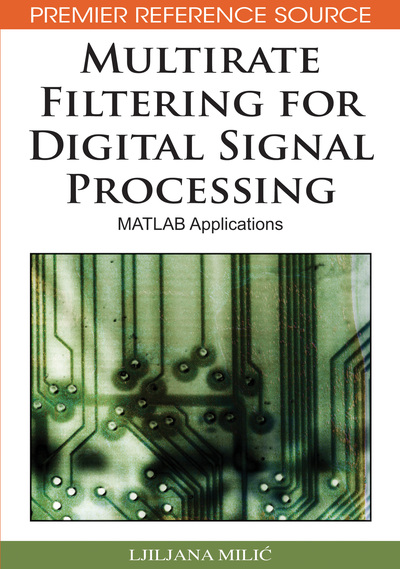# Single-Rate Discrete-Time Signals and Systems: Background Review

Ljiljana Milic (Mihajlo Pupin Institute, Serbia)
DOI: 10.4018/978-1-60566-178-0.ch001
Available
\$33.75
List Price: \$37.50
10% Discount:-\$3.75
TOTAL SAVINGS: \$3.75

## Abstract

This chapter is a concise review of time-domain and transform-domain representations of single-rate discrete-time signals and systems. We consider first the time-domain representation of discrete-time signals and systems. The representation in transform domain comprises the discrete-time Fourier transform (DTFT), the discrete Fourier transform (DFT), and the z-transform. The basic realization structures for FIR and IIR systems are briefly described. Finally, the relations between continuous and discrete signals are given.
Chapter Preview
Top

## Introduction

This chapter is a concise review of time-domain and transform-domain representations of single-rate discrete-time signals and systems. We consider first the time-domain representation of discrete-time signals and systems. The representation in transform domain comprises the discrete-time Fourier transform (DTFT), the discrete Fourier transform (DFT), and the z-transform. The basic realization structures for FIR and IIR systems are briefly described. Finally, the relations between continuous and discrete signals are given.

### DISCRETE-TIME SIGNALS

A signal is a function of at least one independent variable. In this book, we assume that the independent variable is time even in cases where the independent variable is a quantity other than time.

We define a continuous-time signal, xc(t), as a signal that exists at every instant of time t. A continuous-time signal with a continuous amplitude is also called an analog signal. The independent variable t is a continuous variable and xc(t) can assume any value over a continuous range of numbers.

A discrete-time signal is a sequence of numbers denoted as {x[n]}, where n is said to be the time index, and x[n] denotes the value of the nth element in the sequence. A discrete-time signal is called a discrete signal. The quantity x[n] is also called the sample value, and its time index n is called the sample index. The quantity x[n] can take any value over some continuous range of numbers, xminx[n] ≤ xmax.

Discrete signals can be defined only for integer values of n from an interval N1nN2. When the sample values of the sequence {x[n]} are represented as binary numbers using a final number of bits, the signal {x[n]} is a digital signal.

The length of the sequence is defined as N ≤ N2N1 + 1. The sequence {x[n]} is a finite-length sequence if N is of a finite length; otherwise, {x[n]} is an infinite-length sequence.

For the purpose of the analysis, it is useful to represent signals as the combination of basic sequences. The frequently used basic sequences are included in Table 1.

Table 1.
Basic sequences.

In many applications, the discrete-time signal {x[n]} is generated by sampling the continuous-time signal xc(t) at uniform time intervals:. (1)

A time interval T is called a sampling interval or a sampling period, and the reciprocal value,

(2) is a sampling frequency or a sampling rate. In general, the unit of sampling frequency is cycles per second, and when T is given in seconds [s], FT can be expressed in hertz [Hz].

## Complete Chapter List

Search this Book:
Reset